## TyroCity

Physics XI Notes for Physics Notes

Posted on • Updated on

# Addition Of Vectors By Rectangular Components Method

Introduction to rectangular component method

Rectangular component method of addition of vectors is the simplest method to add a number of vectors acting in different directions.

Details of method
Consider two vectors: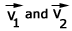making angles q1 and q2 with +ve x-axis respectively.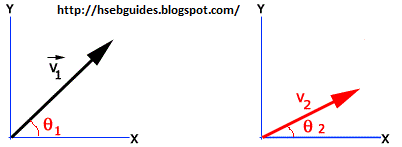Step 1: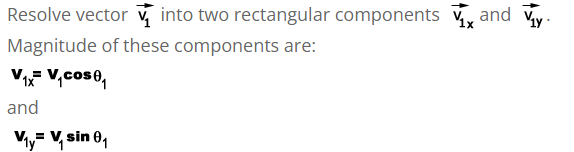Step 2: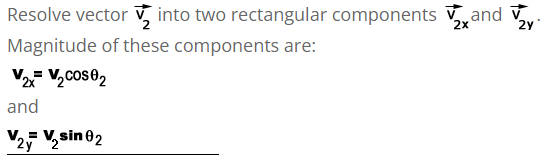Step 3: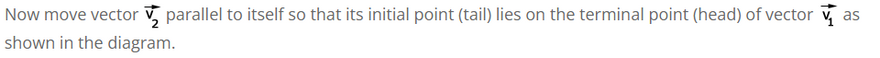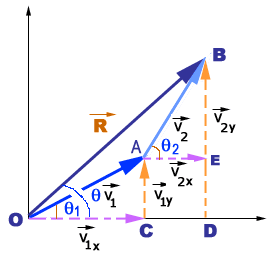Representative lines of v1 and v2 are OA and OB respectively. Join O and B which is equal to resultant vector of v1 and v2.

Step 4:

Resultant vector along X-axis can be determined as: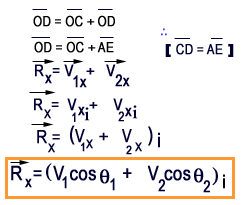Step 5:

Resultant vector along Y-axis can be determined as: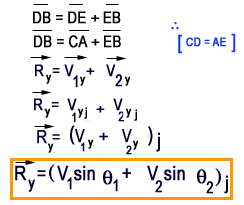Step 6:

Now we will determine the magnitude of resultant vector.
In the right angled triangle DBOD: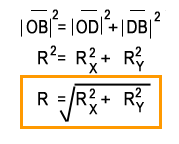Step 7:

Finally, the direction of resultant vector will be determined.
Again in the right angled triangle DBOD: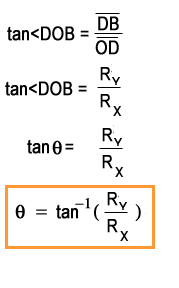Where q is the angle that the resultant vector makes with the positive X-axis.
In this way we can add a number of vectors in a very easy manner.
This method is known as addition of vectors by rectangular components method.